On the meadow grazing horses, cows and sheep, together less than 200. If cows were 45 times more, horses 60 times more and sheep 35 times more than there are now, their numbers would equall. How many
horses, cows and sheep are on the meadow together?

Result

x1 =  85
x2 =  170

#### Solution:

x = a+b+c < 200
b = 45/60 a
c = 45/35 a
a + 45/60 a + 45/35 a < 200
LCD(11, 4560, 4535) = LCM(1, 60, 35) = 22×3×5×7 = 420
11 = 420420
4560 = 315420
4535 = 540420

420 k + 315 k + 540 k < 84000
1275 k < 84000
17 k < 1120

x = 56+42+72 = 170
x = 28+21+36 = 85

Leave us a comment of this math problem and its solution (i.e. if it is still somewhat unclear...):Be the first to comment!#### Following knowledge from mathematics are needed to solve this word math problem:

Do you want to calculate least common multiple two or more numbers? Need help calculate sum, simplify or multiply fractions? Try our fraction calculator.

## Next similar math problems:

1. Lord Ram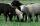When lord Ram founded the breed white sheep was 8 more than black. Currently white sheep are four times higher than at the beginning and black three times more than at the beginning. White sheep is now 42 more than the black. How many white and black sh
2. Mouse Hryzka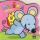Mouse Hryzka found 27 identical cubes of cheese. She first put in a large cube out of them and then waited for a while before the cheese cubes stuck together. Then from every wall of the big cube she will eats the middle cube. Then she also eats the cube
3. TV transmitterThe volume of water in the rectangular swimming pool is 6998.4 hectoliters. The promotional leaflet states that if we wanted all the pool water to flow into a regular quadrangle with a base edge equal to the average depth of the pool, the prism would have.
4. Z9-I-4Kate thought a five-digit integer. She wrote the sum of this number and its half at the first line to the workbook. On the second line wrote a total of this number and its one fifth. On the third row she wrote a sum of this number and its one nines. Fi
5. MO SK/CZ Z9–I–3John had the ball that rolled into the pool and it swam in the water. Its highest point was 2 cm above the surface. Diameter of circle that marked the water level on the surface of the ball was 8 cm. Determine the diameter of John ball.
6. Chamber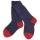In the chamber light is broken and all from it must be taken at random. Socks have four different colors. If you want to be sure of pulling at least two white socks, we have to bring them out 28 from the chamber. In order to have such certainty for the pai
7. Z9–I–1In all nine fields of given shape to be filled natural numbers so that: • each of the numbers 2, 4, 6 and 8 is used at least once, • four of the inner square boxes containing the products of the numbers of adjacent cells of the outer square, • in the cir
8. Mr. ZucchiniMr. Zucchini had a rectangular garden whose perimeter is 28 meters. Content area of the garden filled just four square beds, whose dimensions in meters are expressed in whole numbers. Determine what size could have a garden. Find all the possibilities and
9. Octahedron - sumOn each wall of a regular octahedron is written one of the numbers 1, 2, 3, 4, 5, 6, 7 and 8, wherein on different sides are different numbers. For each wall John make the sum of the numbers written of three adjacent walls. Thus got eight sums, which also.
10. Pentagon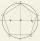Within a regular pentagon ABCDE point P is such that the triangle is equilateral ABP. How big is the angle BCP? Make a sketch.
11. Square gridSquare grid consists of a square with sides of length 1 cm. Draw in it at least three different patterns such that each had a content of 6 cm2 and circumference 12 cm and that their sides is in square grid.
12. Hexagon - MOThe picture shows the ABCD square, the EFGD square and the HIJD rectangle. Points J and G lie on the side CD and is true |DJ|
13. MO - trianglesOn the AB and AC sides of the triangle ABC lies successive points E and F, on segment EF lie point D. The EF and BC lines are parallel and is true this ratio FD:DE = AE:EB = 2:1. The area of ABC triangle is 27 hectares and line segments EF, AD, and DB se
14. Amazing numberAn amazing number is name for such even number, the decomposition product of prime numbers has exactly three not necessarily different factors and the sum of all its divisors is equal to twice that number. Find all amazing numbers.
15. Christmas Day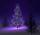In leap years was 53 Sundays. On what day of the week fell to Christmas Day?
16. MO circlesJuro built the ABCD square with a 12 cm side. In this square, he scattered a quarter circle that had a center at point B passing through point A and a semicircle l that had a center at the center of the BC side and passed point B. He would still build a ci
17. Trapezoid MO-5-Z8ABCD is a trapezoid that lime segment CE divided into a triangle and parallelogram as shown. Point F is the midpoint of CE, DF line passes through the center of the segment BE and the area of the triangle CDE is 3 cm2. Determine the area of the trapezoid A